﻿ Quantum Computing 101: What is a Quantum Computer?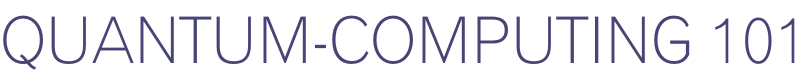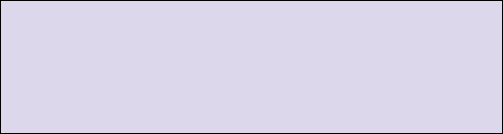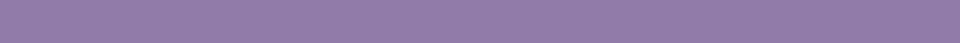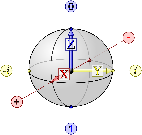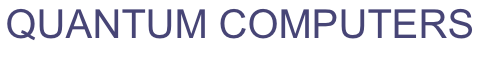# HOW DOES A QUANTUM COMPUTER WORK?

Every computer we interact with in our everyday lives runs on bits. These bits can have values that are either 0 or 1.

It may be difficult to believe, but all of the computations we do on our computers are done with gates where each gate computes a logical operation on the bits.

Computation can be represented in a schematic where bits are wires and gates are different shapes.

There are special gates or groups of gates called "universal gates." The most common universal gates are NAND (and gate followed by a logical not) and NOR (or gate followed by a logical not) gates. A table of input and outputs for a NAND gate (along with all other gates) can be represented in a truth table.

We call the value of a bit a "state." Since the states of bits are deterministic, the computers we use are run through classical physics.

Indeed, many of us learned this type of physics in high school. However, a quantum computer seeks to harness a completely different type of physics called quantum mechanics. Instead of using bits, a quantum computer uses qubits. These qubits are able to take on a superposition of states. Instead of binary computation, a quantum computer is therefore able to encode infinitely many states into a single qubit.

The state of a classical bit would be written in Dirac notation in the computational basis as |0> or |1>.

However, a qubits state can be written as a superposition of states and can be written as a|0> + b|1>.

The state now has a probability of being measured as either a |0> or a |1>. These probabilities are encoded in the constants a and b. Each of these states live in a Hilbert space. This simply means that the states can be written as vectors where the length and angle of the state can be measured (although the length will always be 1 as we can see from the state written above).

To picture these states in three-dimensional space, they can be depicted as a point on the surface of a sphere, called the Bloch sphere.Quantum computers also use gates to perform operations. These gates must be unitary. This ensures that the length of any state during computation is preserved and is always 1 meaning that it stays on the surface of the Bloch sphere.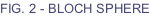While the truth table for some quantum gates, such as the Taffoli gate, can completely specify the gate - for many quantum gates, the truth table cannot. This is because all possible inputs and outputs cannot be enumerated.

Therefore, in quantum computing, gates are described by how they operate on qubits by using matrices.

Quantum computers are very similar to the computers we use in our everyday lives. Quantum-computers simply use bits rather than qubits and quantum gates rather than classical gates. Additionally quantum computers require one more tool - they must be able to measure the qubit.

We can think of this as an experimentalist, who is external to the system observing the internal state of the system. When this happens, we perform an operation to the internal system, which is not unitary. The most basic measurement device is a projection measurement, where a state is "collapsed" into either a |0> or a |1>. The result is probabilistic depending on the weights a and b, which are described above.

As a result, the final state of the qubit is a binary |0> or |1>, identical to a classical bit. So what then is the advantage of a quantum computer if the end result is classical?

The advantage is the flexibility of the computations that can take place before we measure.

PHYSICS REFRESHER:  Superposition means that the net response in all linear systems (for a given time and location) to two or more stimuli - is equivalent to the sum of the responses to the stimuli had they happened individually.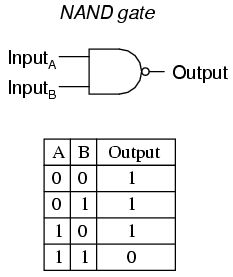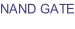IBM’s 4 qubit device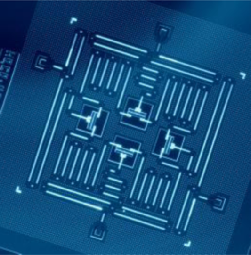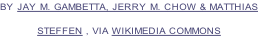The most common type of universal gate for quantum computing is called the Toffoli gate, a CCNot gate (to be exact, for the Toffoli gate to be universal, we must be able to add ancillary bits to the initialized quantum system).

The main difference between a quantum gate and classical gate is that a classical gate can only create a state |0> or |1>, but a quantum gate can create a superposition of states.